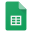Fundamental Basics

forBelow are a few of the most fundamental concepts that all amateur radio operators should have seared into their brains. Familiarity with these concepts is required to earn the Technician Class license, and they will be used throughout your ham career.

Ohm's LawPower CalculationOhm's & Power Calculation CombinedSine Wave

Relationship between Frequency and WavelengthFrequency - Number of cycles completed in one second. Measured in Hertz.

(Above example = 2 Hz.)

Wavelength - Distance traveled in one cycle. Measured in meters.

Period - Amount of time required for one cycle. Measured in seconds.

Amplitude - Strength of the signal; height of the sine wave.

Usually measured in volts or amperes.

RMS = Root Mean Square = the value of the constant direct current that would produce

the same power dissipation in a resistive load.

Frequency - Wavelength ConversionWhere:

λ = Greek letter Lambda = Wavelength in meters

f = Frequency in megaHertz

C = 300 = Approximate speed of light, in millions of meters per second

Note: This conversion is approximate. See the Frequency and Wavelength Conversions section of our Constants and Formulas page for more exact information.

See our Electromagnetic Spectrum page for more details.RF Spectrum

Decibels

dB = 10 log10(P1/P2)

Decibels

3 dB

6

9

10

12

15

18

21

Watts (+/-)

x 2

4

8

10

16

32

64

128

Example: Increasing the power by 9 dB is equal to multiplying the watts by 8.

See the Power Conversions section of our Constants and Formulas page, and our Decibels page for more information.

Resistor Color Code